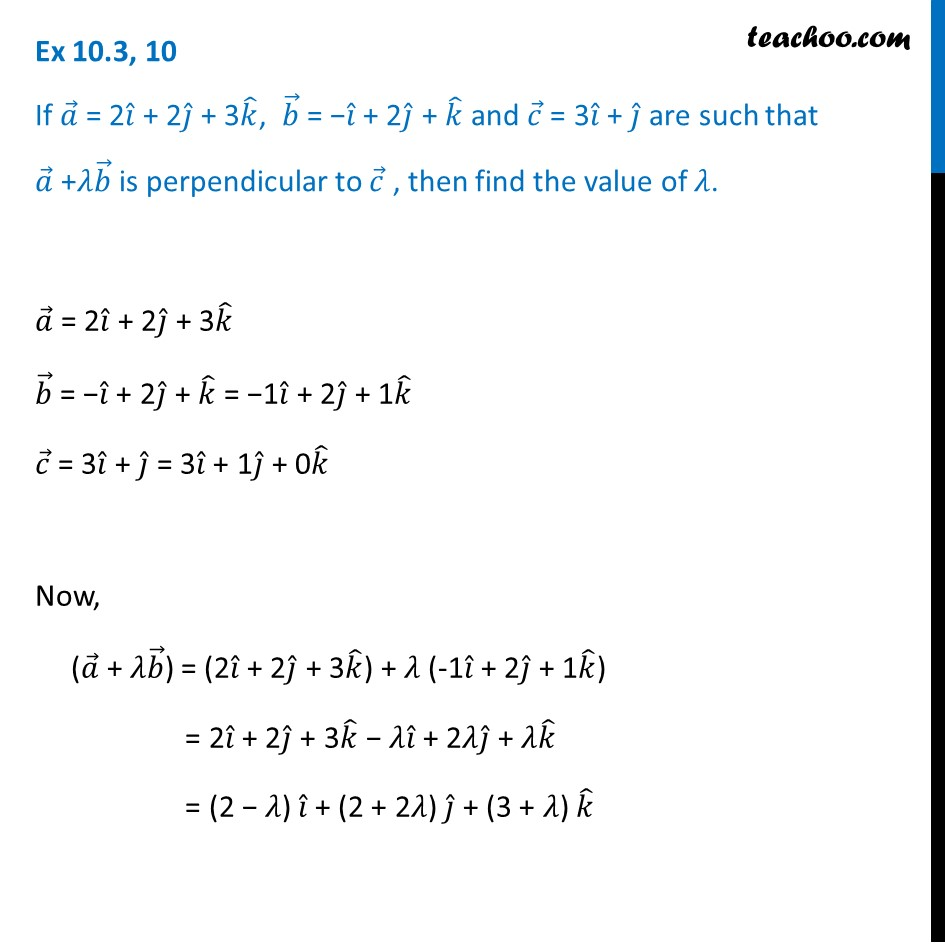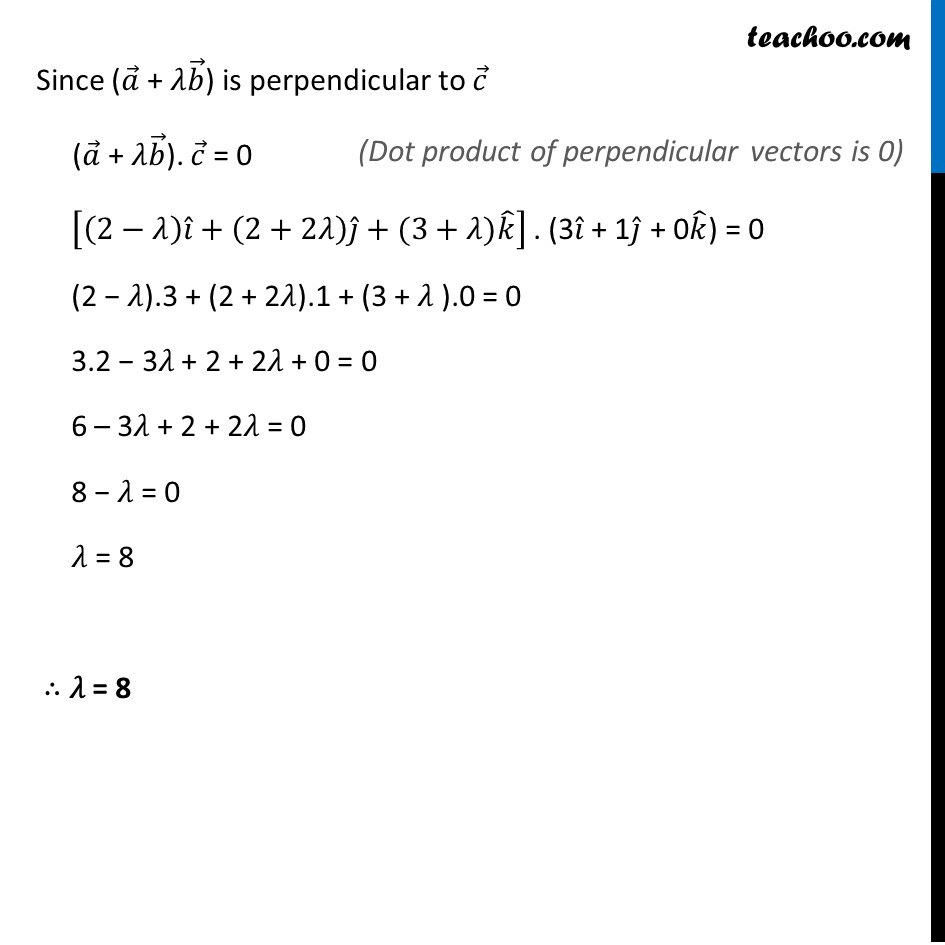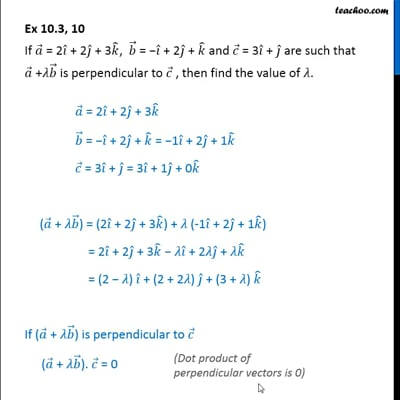Ex 10.3

Chapter 10 Class 12 Vector Algebra
Serial order wiseThis video is only available for Teachoo black users

Introducing your new favourite teacher - Teachoo Black, at only ₹83 per month

### Transcript

Ex 10.3, 10 If 𝑎 ⃗ = 2𝑖 ̂ + 2𝑗 ̂ + 3𝑘 ̂, 𝑏 ⃗ = −𝑖 ̂ + 2𝑗 ̂ + 𝑘 ̂ and 𝑐 ⃗ = 3𝑖 ̂ + 𝑗 ̂ are such that 𝑎 ⃗ +𝜆𝑏 ⃗ is perpendicular to 𝑐 ⃗ , then find the value of 𝜆.𝑎 ⃗ = 2𝑖 ̂ + 2𝑗 ̂ + 3𝑘 ̂ 𝑏 ⃗ = −𝑖 ̂ + 2𝑗 ̂ + 𝑘 ̂ = −1𝑖 ̂ + 2𝑗 ̂ + 1𝑘 ̂ 𝑐 ⃗ = 3𝑖 ̂ + 𝑗 ̂ = 3𝑖 ̂ + 1𝑗 ̂ + 0𝑘 ̂ Now, (𝑎 ⃗ + 𝜆𝑏 ⃗) = (2𝑖 ̂ + 2𝑗 ̂ + 3𝑘 ̂) + 𝜆 (-1𝑖 ̂ + 2𝑗 ̂ + 1𝑘 ̂) = 2𝑖 ̂ + 2𝑗 ̂ + 3𝑘 ̂ − 𝜆𝑖 ̂ + 2𝜆𝑗 ̂ + 𝜆𝑘 ̂ = (2 − 𝜆) 𝑖 ̂ + (2 + 2𝜆) 𝑗 ̂ + (3 + 𝜆) 𝑘 ̂ Since (𝑎 ⃗ + 𝜆𝑏 ⃗) is perpendicular to 𝑐 ⃗ (𝑎 ⃗ + 𝜆𝑏 ⃗). 𝑐 ⃗ = 0 [(2−𝜆) 𝑖 ̂+(2+2𝜆) 𝑗 ̂+(3+𝜆)𝑘 ̂ ] . (3𝑖 ̂ + 1𝑗 ̂ + 0𝑘 ̂) = 0 (2 − 𝜆).3 + (2 + 2𝜆).1 + (3 + 𝜆 ).0 = 0 3.2 − 3𝜆 + 2 + 2𝜆 + 0 = 0 6 – 3𝜆 + 2 + 2𝜆 = 0 8 − 𝜆 = 0 𝜆 = 8 ∴ 𝜆 = 8 (Dot product of perpendicular vectors is 0)